# Self Study - Foundations of Electromagnetic Theory Problem 2-1 (two charged particles hanging on strings)

• Usiia
Usiia
Homework Statement
Two Particles, each of mass m and having charges q and 2q respectively, are suspended from strings of length l from a common point. Find the angle θ that each string makes with the vertical.
Relevant Equations
Coulomb force definition with permittivity variable present.
I'd like someone to check that this solution is right, and if not, give me the point of failure and not the answer.

Thanks!AntSC
Error in your first equation. Otherwise, all the components are there and looks good

•Usiia
Usiia
Thanks! Will take another look and report back!

Usiia
Ok, second try: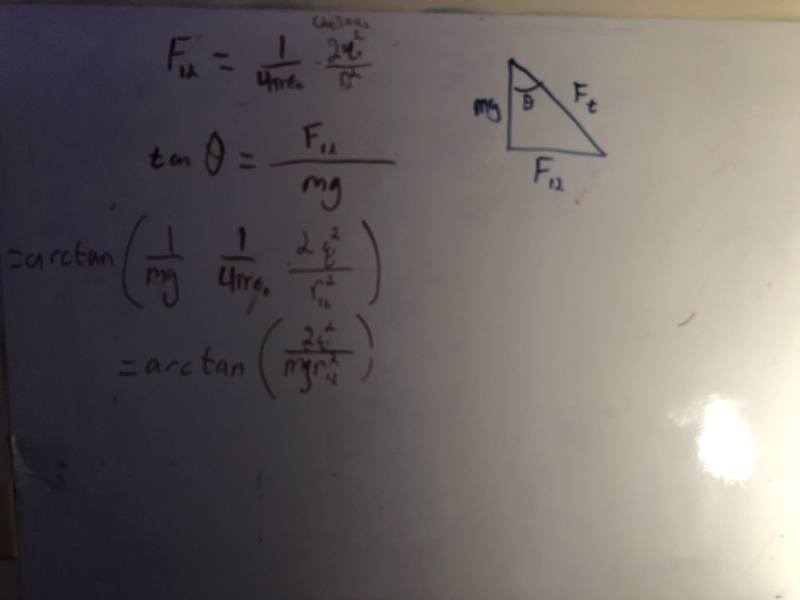Homework Helper
Attachment disappeared !

Usiia
needed a correction, making that now, having camera/file issues.

EDIT: No, I the right one I meant to upload was wrong. One sec.

ok, try number 4: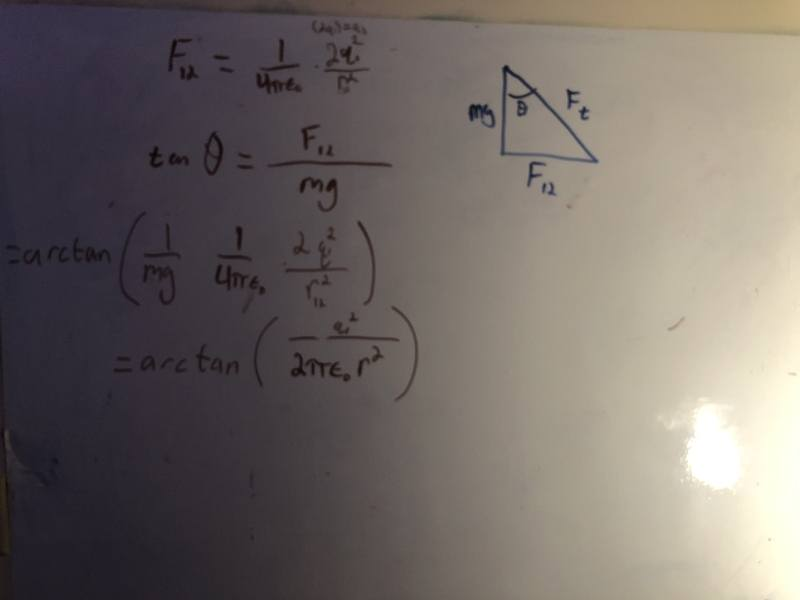Homework Helper
Picture OK now (well, a bit of ##LaTeX## would be a lot better) . What about ##r_{12}## ?

•PhDeezNutz and Usiia
Usiia
Tried using latex, mathjax wouldn't work

Usiia
Also, I'm incredibly good at LaTeX as well as a CS person, but I don't like it for HW type problems. I just get caught up making it prefect looking instead of right.

If it's a space thing, I'll try harder :)

Usiia
So, I left out r_1,2 normalized in this because it wasn't necessary to figure out magnitudes of the force, the direction laterally didn't seem important.

AntSC
needed a correction, making that now, having camera/file issues.

EDIT: No, I the right one I meant to upload was wrong. One sec.

ok, try number 4:

View attachment 294532

Looks good to me. Yes, it was your rearrangement of ##F=mg\tan \theta## that was the issue. Everything else seems good

•Usiia
Usiia
Thank you so much! I'll work on getting the LaTeX working for presenting my answers better and preserving the server storage!

•berkeman
AntSC
Thank you so much! I'll work on getting the LaTeX working for presenting my answers better and preserving the server storage!
There is a thread on the use of Latex for PF if needed.

•Usiia
Usiia
I'm going to work on this a little more because I feel some identities could simplify this result.

Usiia
So I am using an arctan identity

arctan(2mg*pi*permit*r^2/q^2)= -pi/2 - arctan(2mg*pi*permit*r^2/q^2)

and so

-pi/2 - arctan(2mg*pi*permit*r^2/q^2)

EDITED: So, if we took the limit m->0, g->0 or we'd get that this system wants to be at no angle with no force (which is right) and otherwise, q->inf, it's going to be some amount less than 180 degrees.

That interpretation is correct right?

Last edited:
Homework Helper
So arctan(x) = -π/2 - arctan(x)?
Did you mean arctan(x) = π/2 - arctan(1/x)?
You are being very sloppy, as is shown by missing terms out in some of the equations you have posted. This is not the way to get right answers.
EDITED: So, if we took the limit m->0, g->0 or we'd get that this system wants to be at no angle with no force (which is right)
Which is wrong. If m or g = 0, there is no vertical force, so θ = 90°, where the particles are as far apart as possible.

What about r? You are not given r, but you are given l, the length of the string. What is r in terms of l and θ? (This makes things more complicated!)

Usiia

The identity I found had - π/2 and I had inverted the internal expression.

I believed that this was correct with my choice of origin. Also, -π/2 is 0 degrees in the orientation I choose where g points down.

I apologize if I had been sloppy. I am just getting back into this work. I will work on my presentation and draft better.

Usiia
What about r? You are not given r, but you are given l, the length of the string. What is r in terms of l and θ? (This makes things more complicated!)

Figured out the block quote, so yes, I agree and will work on that as well. Thank you!

Homework Helper
Good. Kudos to you for liking my post though it criticised you; that's the way to learn! Good luck.

•Usiia
Usiia
Good. Kudos to you for liking my post though it criticised you; that's the way to learn! Good luck.
I'm sadly aware of how far I've slipped.

Homework Helper
Gold Member
The problem gives you the length of the string ##l## not the separation between the masses. Therefore your answer must be in terms of ##l##, i.e. given ##l## in meters and ##q## in Coulombs, find ##\theta## in radians. @BvU pointed that out in post #7.

•Usiia
Usiia
The problem gives you the length of the string ##l## not the separation between the masses. Therefore your answer must be in terms of ##l##, i.e. given ##l## in meters and ##q## in Coulombs, find ##\theta## in radians. @BvU pointed that out in post #7.
Yes, I see and realize that now. Thank you.

Last edited:
Usiia
So, to start I have two related triangles. One of the forces and one of the lengths.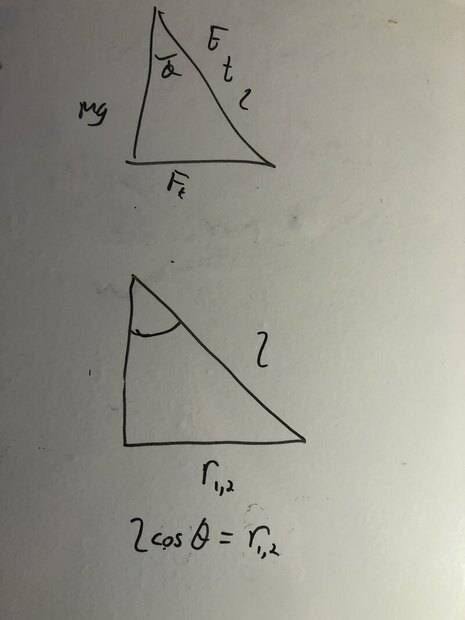$$F_{1,2} \cos \theta - mg \sin \theta = 0$$
$$r_{1,2} = l \cos \theta$$
$$F_{1,2} = \dfrac {1}{4 \pi \epsilon_0} \dfrac {2q^2}{r_{1,2}^2}$$

And then
$$\dfrac{q^2 \cos \theta}{2 \pi \epsilon_0 ( l \cos \theta )^2 } = mgl \sin \theta$$

$$\dfrac{q^2 }{2 \pi \epsilon_0 (\cos \theta l) ^2 } = mgl \sin \theta$$

Is this right so far or should I be treating this like a related rates problem?

Last edited:
Homework Helper
Gold Member
2022 Award
I must admit I don't understand your diagram or your equations. Why not use ##T## for the tension in the string; ##F## for the electrostatic force; and, ##r## for the distance between the masses?

I suggest you show both masses on the diagram, so you can see how ##r## relates to ##\cos \theta## (or, should that be ##\sin \theta##?)

•Usiia
Usiia
I must admit I don't understand your diagram or your equations. Why not use ##T## for the tension in the string; ##F## for the electrostatic force; and, ##r## for the distance between the masses?

I suggest you show both masses on the diagram, so you can see how ##r## relates to ##\cos \theta## (or, should that be ##\sin \theta##?)
I will draw out the masses and take another look at the trig functions, thanks!

Usiia
I will draw out the masses and take another look at the trig functions, thanks!
Ok, here's a more complete diagram.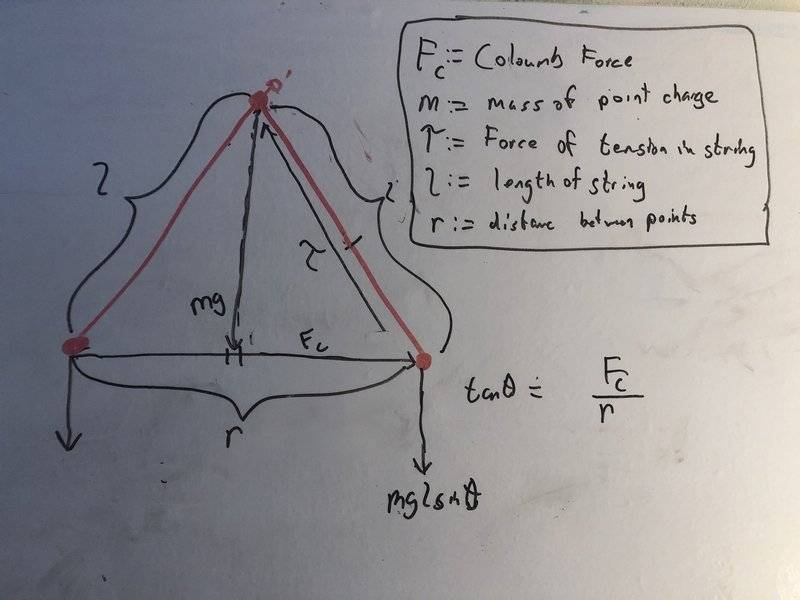ignore the wrong note on tan to the right.

Homework Helper
Gold Member
2022 Award
That doesn't look right. The gravitational force on each mass is simply ##mg##. The same force ##F## acts on each particle (hence the symmetry).

Usiia
You're right. I got caught up in finding out where to place that sin.

•PeroK
Usiia
Is this more accurate?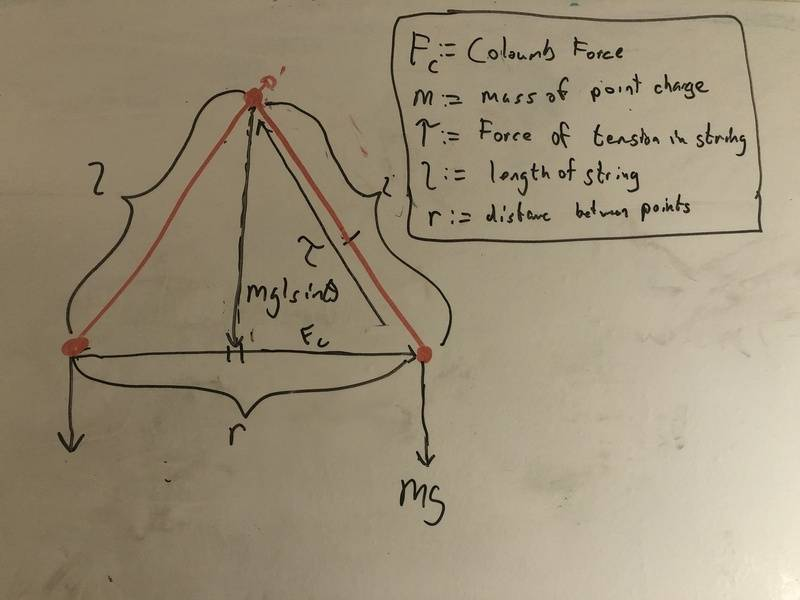Homework Helper
Gold Member
2022 Award
Just to say that I'm getting an "unsolvable" cubic equation in ##\tan \theta##. Does the question expect an exact solution?

•Usiia
Usiia
Just to say that I'm getting an "unsolvable" cubic equation in ##\tan \theta##. Does the question expect an exact solution?
It is a cubic relation.

Usiia
Answer provided that I just found at the back of the book $$\tan^3 \theta ( 1+ \tan^2 \theta ) = \dfrac{q^2}{8 \pi \epsilon_0 mgl^2}$$

Usiia
Answer provided that I just found at the back of the book $$\tan^3 \theta ( 1+ \tan^2 \theta ) = \dfrac{q^2}{8 \pi \epsilon_0 mgl^2}$$
Of course this means nothing to me. I am supposing that this is a related rates problem and that I need more than what I think I have so far in terms of the tangent.

Homework Helper
Gold Member
2022 Award
Of course this means nothing to me. I am supposing that this is a related rates problem and that I need more than what I think I have so far in terms of the tangent.
First, generate the force balancing equations for ##T, F## and ##mg##.

•Usiia
Usiia
First, generate the force balancing equations for ##T, F## and ##mg##.
Need to go to work now, but will come back to this with this approach.

Thank you so much!